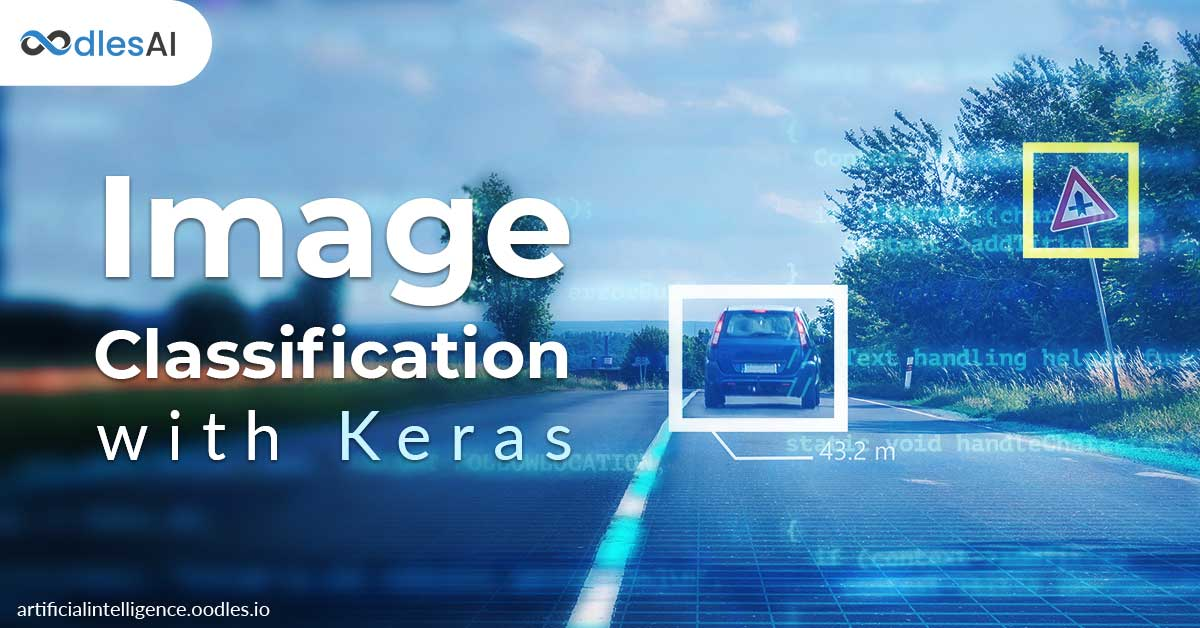# Building Image Classification Model with Keras

#### Harshit Verma | 27th December 2019Artificial intelligence (AI) works on prodigious amounts of data feeds to achieve cognitive abilities. Rich machine learning libraries such as Keras and TensorFlow are contributing to the dynamic artificial intelligence services. Keras and Tensorflow together support model training to build image recognition, deep video analytics, brand monitoring, facial gesture recognition, and other machine learning models.

This post highlights some common operations that you would frequently need in the Keras. First, we will understand how to save the models and use them for prediction later. Also, this post explains how to display the images from a dataset and load images from our systems to predict the class.

## Training and Saving the Model

Training the models is a very slow process, nobody wants to do that every time. Fortunately, we only need to train the model once, save it and then we can load it anytime and use it to predict the new images. Keras saves the models in the .h5 format.

import keras

from keras.datasets import mnist

from keras.layers import Dense

from keras.models import Sequential

from keras.optimizers import SGD

(train_x, train_y) , (test_x, test_y) = mnist.load_data()

#train_x = train_x.astype(‘float32’) / 255

#test_x = test_x.astype(‘float32’) / 255

print(train_x.shape)

print(train_y.shape)

print(test_x.shape)

print(test_y.shape)

train_x = train_x.reshape(60000,784)

test_x = test_x.reshape(10000,784)

train_y = keras.utils.to_categorical(train_y,10)

test_y = keras.utils.to_categorical(test_y,10)

model = Sequential()

model.add(Dense(units=128,activation=“relu”,input_shape=(784,)))

model.add(Dense(units=128,activation=“relu”))

model.add(Dense(units=128,activation=“relu”))

model.add(Dense(units=10,activation=“softmax”))

model.compile(optimizer=SGD(0.001),loss=“categorical_crossentropy”,metrics=[“accuracy”])

model.fit(train_x,train_y,batch_size=32,epochs=10,verbose=1)

accuracy = model.evaluate(x=test_x,y=test_y,batch_size=32)

print(“Accuracy: “,accuracy)

To save model, simply add below after model.fit()

model.save(“mnist-model.h5”)

### Inference

Inference refers to  process of predicting the images using our model.

import keras

from keras.datasets import mnist

from keras.layers import Dense

from keras.models import Sequential

from keras.optimizers import SGD

(train_x, train_y) , (test_x, test_y) = mnist.load_data()

#train_x = train_x.astype(‘float32’) / 255

#test_x = test_x.astype(‘float32’) / 255

print(train_x.shape)

print(train_y.shape)

print(test_x.shape)

print(test_y.shape)

train_x = train_x.reshape(60000,784)

test_x = test_x.reshape(10000,784)

train_y = keras.utils.to_categorical(train_y,10)

test_y = keras.utils.to_categorical(test_y,10)

model = Sequential()

model.add(Dense(units=128,activation=“relu”,input_shape=(784,)))

model.add(Dense(units=128,activation=“relu”))

model.add(Dense(units=128,activation=“relu”))

model.add(Dense(units=10,activation=“softmax”))

model.compile(optimizer=SGD(0.001),loss=“categorical_crossentropy”,metrics=[“accuracy”])

model.load_weights(“mnist-model.h5”)

#model.fit(train_x,train_y,batch_size=32,epochs=10,verbose=1)

#model.save(“mnistmodel.h5”)

accuracy = model.evaluate(x=test_x,y=test_y,batch_size=32)

print(“Accuracy: “,accuracy)

We loaded the parameters of the model from the saved model file and evaluated that function runs prediction over test dataset and returns accuracy of our predictions.

So far, we have demonstrated how to save the models and use them later for prediction. However, this is a comparatively easy and common task. The main task is being able to load a specific image and determine what class it belongs to.

img = test_x

test_img = img.reshape((1,784))

img_class = model.predict_classes(test_img)

prediction = img_class

classname = img_class

print(“Class: “,classname)

Here we just pick a random image. In this case at index 130 from the test set, we create the flatten copy that is reshaped.

Now that we have a prediction, we use Matplotlib to display the image and its predicted class.

img = img.reshape((28,28))

plt.imshow(img)

plt.title(classname)

plt.show()

import keras

from keras.datasets import mnist

from keras.layers import Dense

from keras.models import Sequential

from keras.optimizers import SGD

import matplotlib.pyplot as plt

(train_x, train_y) , (test_x, test_y) = mnist.load_data()

train_x = train_x.reshape(60000,784)

test_x = test_x.reshape(10000,784)

train_y = keras.utils.to_categorical(train_y,10)

test_y = keras.utils.to_categorical(test_y,10)

model = Sequential()

model.add(Dense(units=128,activation=“relu”,input_shape=(784,)))

model.add(Dense(units=128,activation=“relu”))

model.add(Dense(units=128,activation=“relu”))

model.add(Dense(units=10,activation=“softmax”))

model.compile(optimizer=SGD(0.001),loss=“categorical_crossentropy”,metrics=[“accuracy”])

model.load_weights(“mnistmodel.h5”)

img = test_x

test_img = img.reshape((1,784))

img_class = model.predict_classes(test_img)

prediction = img_class

classname = img_class

print(“Class: “,classname)

img = img.reshape((28,28))

plt.imshow(img)

plt.title(classname)

plt.show()

But, what if we want to upload an image that is not included in the test set? For this test, please save the image below to your system and copy it into the directory where your python file resides.

You might notice the few new things here. First, we imported the image from keras.preprocessing.

img = image.load_img(path=“testimage.png”,grayscale=True,target_size=(28,28,1))

img = image.img_to_array(img)

In the first line, we loaded the image from disk and specified that it should be resized to 28 x 28 x 1. Remember that the dimension of the original mnist images, so its good that we keep things constant.

Next, we converted the image into an array of pixels, and we are good to go.

Run it and check the result yourself.

For more assistance in building function-specific image classification and prediction models, talk to the Oodles AI team today.

### About Author#### Harshit Verma

Harshit is a bright Web Developer with expertise in Java and Spring framework and ORM tools Hibernate.

No Comments Yet.

Request For Proposal

Sending message..

Chat With Us